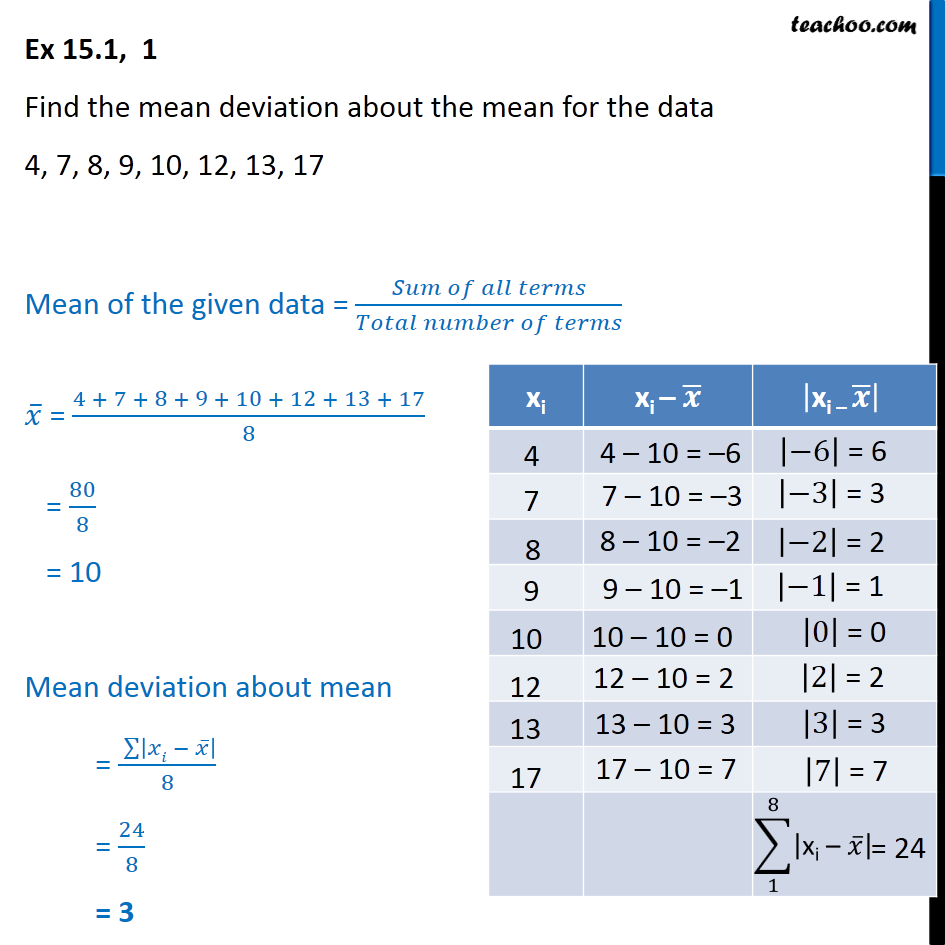Mean deviation about mean - Ungrouped

Chapter 13 Class 11 Statistics
Concept wiseLearn in your speed, with individual attention - Teachoo Maths 1-on-1 Class

### Transcript

Ex 13.1, 1 Find the mean deviation about the mean for the data 4, 7, 8, 9, 10, 12, 13, 17 We use the formula Mean of the data = Sum of all terms / Total numbers of terms Mean = 4 + 7 + 8 + 9 + 10 + 12 + 13 + 17 / 8 = 80/8 = 10 We also find mean deviation about mean = Sum | x - Mean| /8 = 24/8 = 3